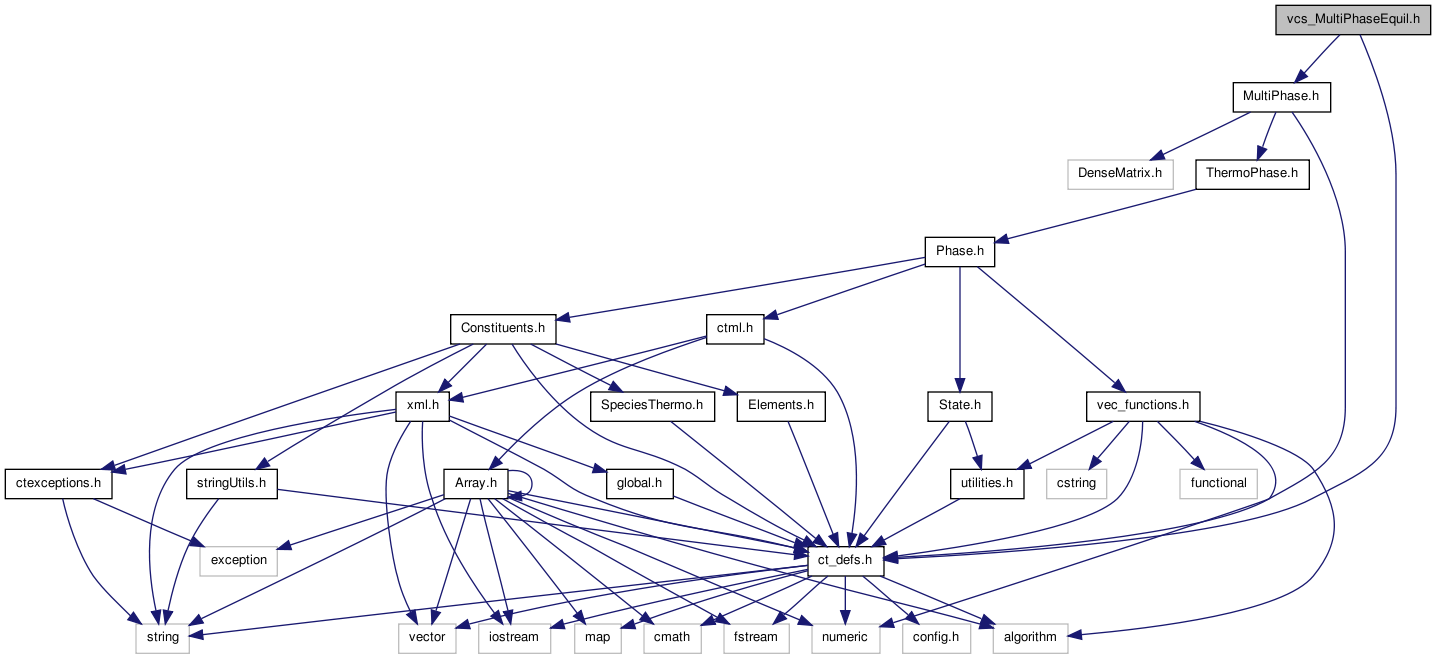# vcs_MultiPhaseEquil.h File Reference

Interface class for the vcsnonlinear solver. More...

`#include "ct_defs.h"`
`#include "MultiPhase.h"`
Include dependency graph for vcs_MultiPhaseEquil.h:Go to the source code of this file.

## Classes

class  vcs_MultiPhaseEquil
Cantera's Interface to the Multiphase chemical equilibrium solver. More...

## Namespaces

namespace  Cantera

Namespace for the Cantera kernel.

## Functions

int vcs_equilibrate (thermo_t &s, const char *XY, int estimateEquil=0, int printLvl=0, int solver=-1, doublereal rtol=1.0e-9, int maxsteps=5000, int maxiter=100, int loglevel=-99)
Set a single-phase chemical solution to chemical equilibrium.
int vcs_equilibrate (MultiPhase &s, const char *XY, int estimateEquil=0, int printLvl=0, int solver=2, doublereal rtol=1.0e-9, int maxsteps=5000, int maxiter=100, int loglevel=-99)
Set a multi-phase chemical solution to chemical equilibrium.
int vcs_equilibrate_1 (MultiPhase &s, int ixy, int estimateEquil=0, int printLvl=0, int solver=2, doublereal rtol=1.0e-9, int maxsteps=5000, int maxiter=100, int loglevel=-99)
Set a multi-phase chemical solution to chemical equilibrium.
int vcs_Cantera_to_vprob (Cantera::MultiPhase *mphase, VCSnonideal::VCS_PROB *vprob)
Translate a MultiPhase object into a VCS_PROB problem definition object.
int vcs_Cantera_update_vprob (Cantera::MultiPhase *mphase, VCSnonideal::VCS_PROB *vprob)
Translate a MultiPhase information into a VCS_PROB problem definition object.

## Detailed Description

Interface class for the vcsnonlinear solver.

Definition in file vcs_MultiPhaseEquil.h.

Generated by1.6.3